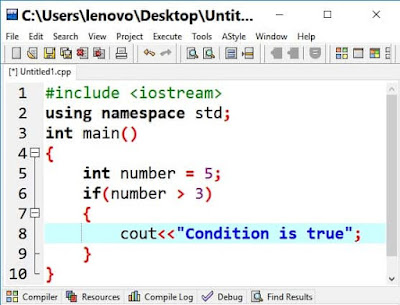## If Statement

In C/C++, the statement is used to execute a particular block of code if a certain condition is true. Three types of statements are used in C/C++
• If statement
• If-else statement
• Switch statement

## Syntax of if statement

if(condition)
{
body code
}

Here body code will only be executed if the condition is true

The below code will check a variable number whether less than 5 or not. As the number is 4 so condition will be true and the body code will be executed. In the body, the output is displayed to users that number is less than 5.

int number = 4;
if(number < 5)
{
cout<<"Less then 5";
}

Write a Program to use if statement in C/C++If statement
OutputIf statement output